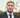# Compound interest php function

April 01, 2010

Something that will crop up at some point in your programming lifespan is a little bit of compound interest.

Well its very easy to work it out. The below example does so over a 7 day period at 1.8% interest per day.

``` function compound(\$amount,\$time,\$rate){ for(\$i=0;\$i<\$time;\$i++){ \$amount = \$amount + round(\$amount*\$rate); } return \$amount; } \$compound = compound(1000000,7,0.018); echo number_format(\$compound); ```